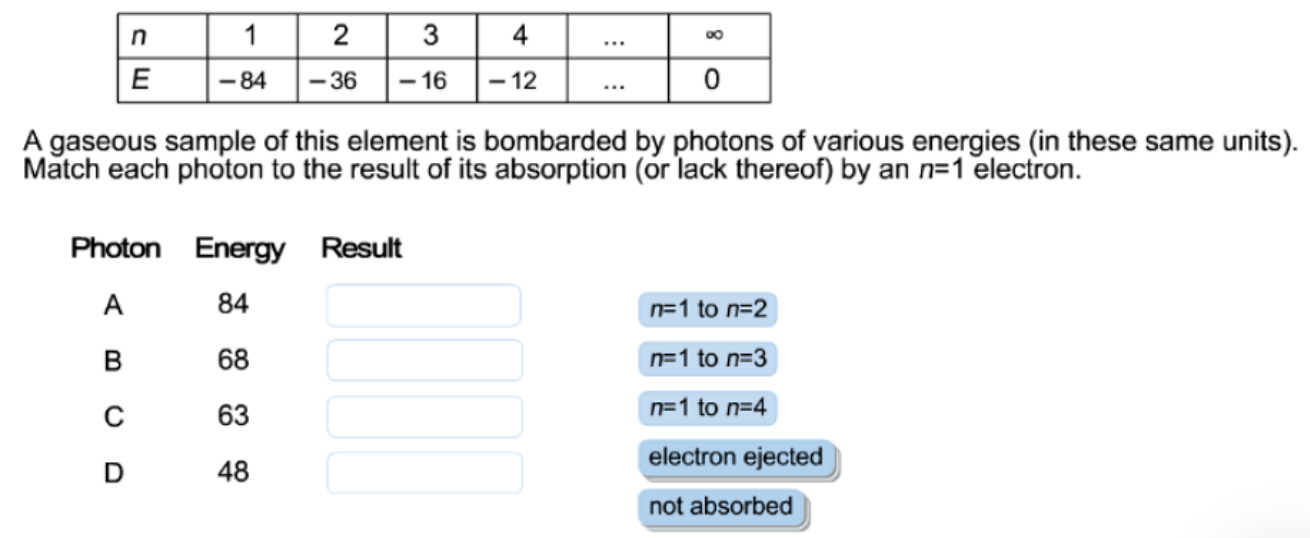# Problem: The energies, E, for the first few states of an unknown element are shown here in arbitrary units.

###### FREE Expert Solution

Recall that starting from n = 1, the distance between each energy level gets smaller as shown below:Emission is a transition process from a higher energy level to a lower energy level

Recall that the energy of a photon is given by:We can see that energy and frequency are directly proportional. The energy in a transition also depends on the distance between the energy levels.

80% (152 ratings)###### Problem Details

The energies, E, for the first few states of an unknown element are shown here in arbitrary units.Frequently Asked Questions

What scientific concept do you need to know in order to solve this problem?

Our tutors have indicated that to solve this problem you will need to apply the Bohr Model concept. You can view video lessons to learn Bohr Model. Or if you need more Bohr Model practice, you can also practice Bohr Model practice problems.

What professor is this problem relevant for?

Based on our data, we think this problem is relevant for Professor Rosensweig & Weitz's class at NU.